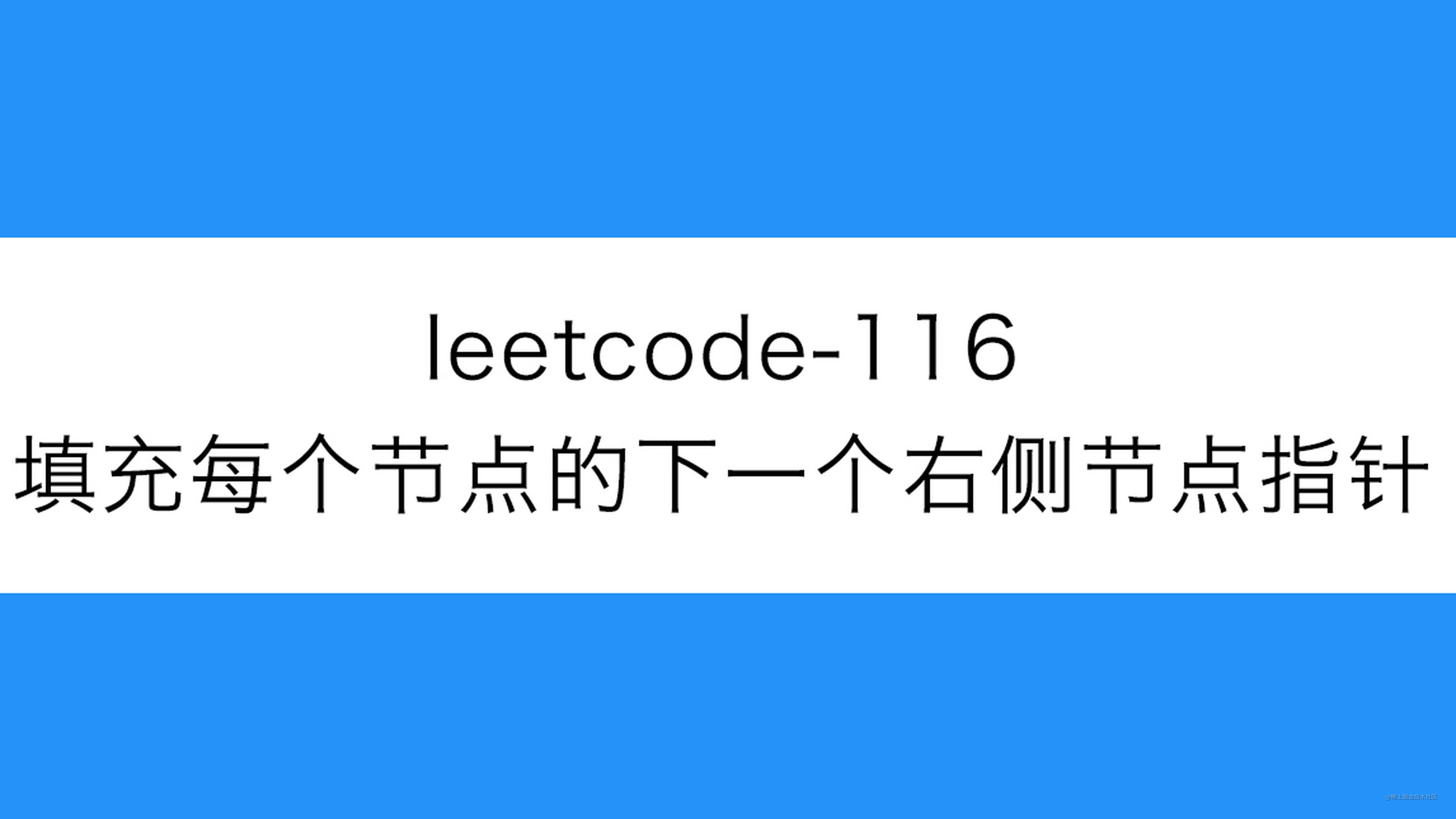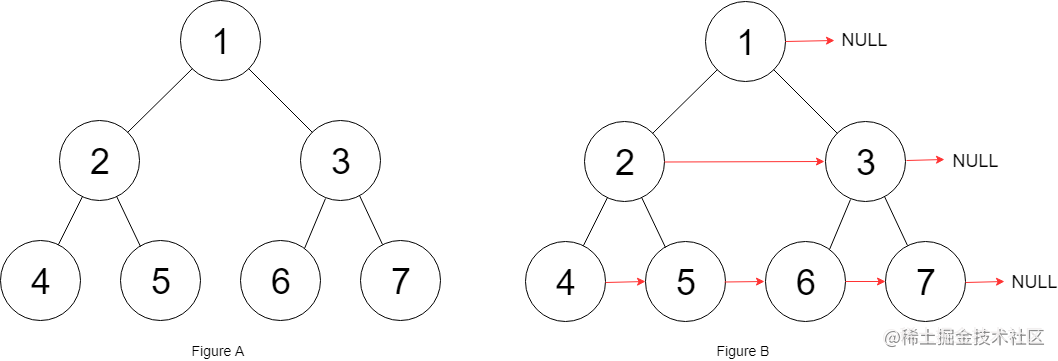# leetcode-116-填充每个节点的下一个右侧节点指针``````struct Node {
int val;
Node *left;
Node *right;
Node *next;
}``````输入： root = [1,2,3,4,5,6,7]

``````输入： root = []

• 树中节点的数量在 `[0, 212 - 1]` 范围内
• `-1000 <= node.val <= 1000`

• 你只能使用常量级额外空间。
• 使用递归解题也符合要求，本题中递归程序占用的栈空间不算做额外的空间复杂度。

### 解题思路-基础

1. 把每一层的节点从左到右收集到一起
2. 把收集到的每一层节点按顺序连接

### 代码实现

``````var connect = function(root) {
if(root === null){
return null
}

// 前序遍历按层收集节点
const collection = []
function preorder(node,deep){
if(node === null){
return
}
if(!collection[deep]){
collection[deep] = []
}
collection[deep].push(node)
preorder(node.left,deep+1)
preorder(node.right,deep+1)
}
preorder(root,0)

// 处理每一层节点的 next指针
for(let i = 0;i<collection.length;i++){
const list = collection[i]
for(let j = 0;j<list.length-1;j++){
list[j].next = list[j+1]
}
}

return root
};

### 代码实现

``````var connect = function(root) {
if(root === null){
return null
}
// 预计总节点数
let totalNum = 1
// 节点队列
const queue = [root]
// 已处理节点数量
let num = 0
// 上一个节点
let pre = null
while(queue.length){
const current = queue.shift()
num++

// 如果当前是这一层最后一个，更新 totalNum
if(num === totalNum){
totalNum *= 2
}else{
// 否则连接next指针
pre.next = current
}

pre = current

if(current.left){
queue.push(current.left)
}
if(current.right){
queue.push(current.right)
}
}

return root
};

### 代码实现

``````var connect = function(root) {
if (root === null) {
return null
}

// 初始化待处理层最左侧节点
let leftmost = root
while (leftmost.left !== null) {
let current = leftmost
// 连接当前节点的左右子节点
while (current !== null) {
current.left.next = current.right
// 如果当前节点的next节点存在
if (current.next != null) {
// 连接当前节点的右子节点和next指针的左子节点
current.right.next = current.next.left
}
// 继续处理当前层节点
current = current.next
}
// 处理下一层节点
leftmost = leftmost.left
}

return root
};